# Featurizations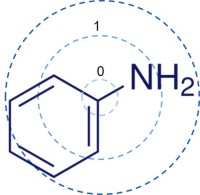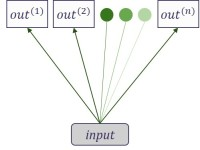Standard classification model by applying logistic function to weighted linear combination of input features.
Molecule  is decomposed into segments of variable sizes, all originated from heavy atoms(C, N, O).
All segment are then assigned with  unique identifiers, which are hashed together into a fixed length binary fingerprint.
Classification
Deterministic
Deterministic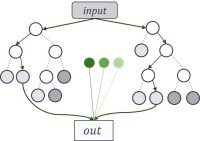Standard classification and regression method based on an ensemble of decision trees, each trained on a different subsampled version of the original dataset.
Regression
Deterministic
Classification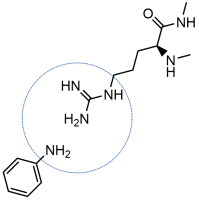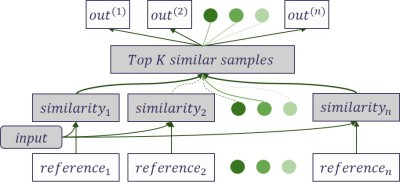Grid Featurizer, initially built for PDBbind,  relies on detailed structures of protein-ligand pair to summarize inter-molecular forces. It incorporates fingerprints of both proteins and ligands, as well as an enumeration of salt bridges, hydrogen bonding, etc.
Refined K-nearest neighbour classifier. Using the hypothesis that compounds with similar substructures have similar functionality, it makes prediction by combining labels from the top-K compounds most similar to the sample.
Classification
Deterministic
Deterministic
3D Coordinates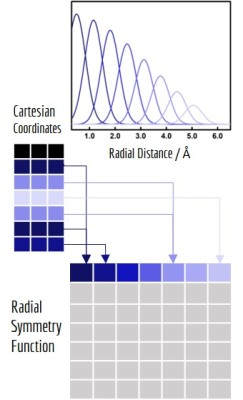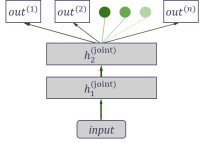Symmetry functions is another encoding of Cartesian coordinates which focuses on preserving the rotational and permutation symmetry of the system. It introduces a series of radial and angular symmetry functions  with different distance and angle cutoffs.
Standard neural network prediction method designed for multitask settings. Input features are processed through multiple shared fully-connected layers and then fed into separate linear classifiers/regressors for each different task. In case of single task dataset, it will become vanilla neural network model.
Deterministic
3D Coordinates
Regression
Deterministic
Classification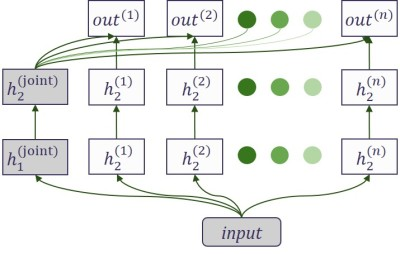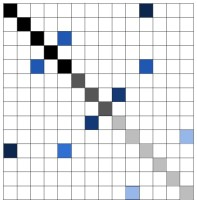Coulomb Matrix encodes nuclear charges and corresponding Cartesian coordinates into a matrix, with diagonal elements representing nuclear charges and off-diagonal elements representing Coulomb repulsions.
A modified version of multitask network designed for uncorrelated tasks. Based on the structure of multitask network, it adds "bypass" layers directly connecting input features and each individual task, hence increasing explanatory power in case of unrelated variations in the sample.

Deterministic
3D Coordinates
Regression
Deterministic
Classification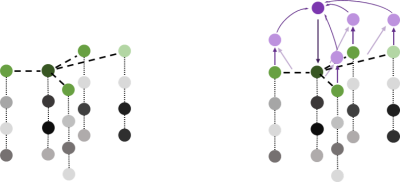Adaptable extension of the Coulomb Matrix featurizer. Nuclear charges(atom types) are mapped to feature vectors, which are further updated based on distance matrix and neighbour atoms. Final states of all atoms' feature vectors are mapped to the outputs and then summed to predict molecular properties.
Regression
3D Coordinates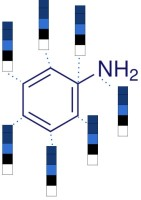Molecule is represented by a neighbout list and a set of initial feature vectors , each corresponding to a single atom,. Feature vector summarizes the atom's local chemical environment,  including atom-types, hybridization types and valence structures.
A learnable version of circular fingerprint which replaces fixed hash functions by differentiable network layers. In graph convolutional models, molecules are treated as undirected graphs: atoms as nodes and bonds as edges. Each convolutional layer will extend the feature vector of the central atom by applying convolutional functions(network layer) on itself and its neighbours(other nodes connected by edges).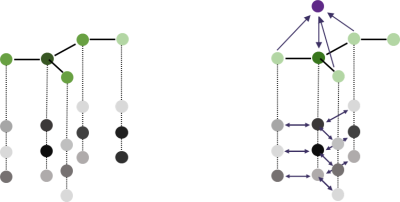Variable
Regression
Variable
Classification
An alternate of graph-based method that applies to directed graphs. The model regards each molecule as a set of directed acyclic graphs, each originated from a different atom. Results from all possible graphs of a molecule are calculated and averaged to yield molecular-level properties.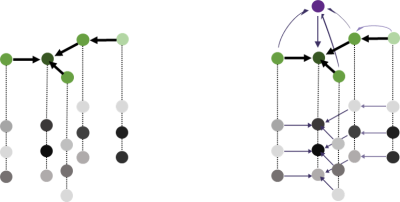Regression
Variable
Classification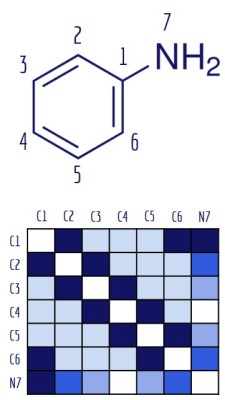A similar adaptive graph-based model that treats molecules as undirected graphs. Instead of doing convolution locally(central atom and neighbour atoms), it applies global convolutions to central atom and all other atoms in the molecule, together with their corresponding pair features.

With the same feature vectors for atoms as Graph convolutions featurizer, Weave featurizer elaborates the neighbour list as a matrix of pair feature vectors, each representing the connectivity and distance between a pair of atoms.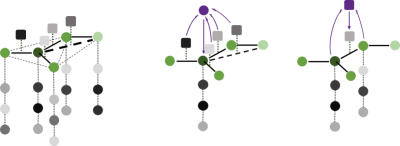Regression
Variable
Variable
Classification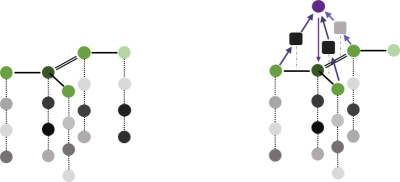Message passing neural network(MPNN) is a generalized graph-based model. Its prediction process is separated into two phases: message passing phase(an edge dependent neural network) and readout phase(seq2seq model for sets).
Regression
Variable
Classification